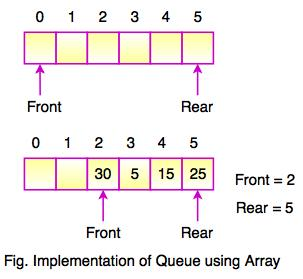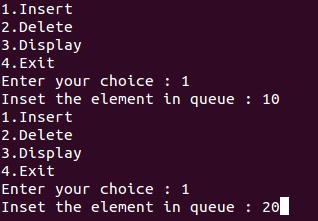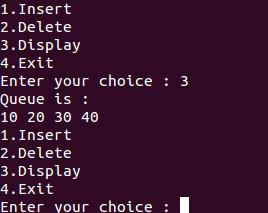# Queue in Data Structure

## What is Queue?

• Queue is a linear data structure where the first element is inserted from one end called REAR and deleted from the other end called as FRONT.
• Front points to the beginning of the queue and Rear points to the end of the queue.
• Queue follows the FIFO (First - In - First Out) structure.
• According to its FIFO structure, element inserted first will also be removed first.
• In a queue, one end is always used to insert data (enqueue) and the other is used to delete data (dequeue), because queue is open at both its ends.
• The enqueue() and dequeue() are two important functions used in a queue.## Operations on Queue

Following are the basic operations performed on a Queue.

OperationsDescription
enqueue()This function defines the operation for adding an element into queue.
dequeue()This function defines the operation for removing an element from queue.
init()This function is used for initializing the queue.
FrontFront is used to get the front data item from a queue.
RearRear is used to get the last item from a queue.

## Queue Implementation

• Array is the easiest way to implement a queue. Queue can be also implemented using Linked List or Stack.

•• In the above diagram, Front and Rear of the queue point at the first index of the array. (Array index starts from 0).
• While adding an element into the queue, the Rear keeps on moving ahead and always points to the position where the next element will be inserted. Front remains at the first index.

#### Example: Program to implement a queue using Array

#include <stdio.h>
#define MAX 50
int queue_array[MAX];
int rear = - 1;
int front = - 1;
main()
{
int choice;
while (1)
{
printf("1.Insert \n");
printf("2.Delete\n");
printf("3.Display \n");
printf("4.Exit \n");
scanf("%d", &choice);
switch (choice)
{
case 1:
insert();
break;
case 2:
delete();
break;
case 3:
display();
break;
case 4:
exit(1);
default:
printf("Inavlid choice \n");
} /*End of switch*/
} /*End of while*/
} /*End of main()*/
insert()
{
if (rear == MAX - 1)
printf("Queue Overflow \n");
else
{
if (front == - 1)
/*If queue is initially empty */
front = 0;
printf("Inset the element in queue : ");
rear = rear + 1;
}
} /*End of insert()*/
delete()
{
if (front == - 1 || front > rear)
{
printf("Queue Underflow \n");
return ;
}
else
{
printf("Deleted Element is : %d\n", queue_array[front]);
front = front + 1;
}
} /*End of delete() */
display()
{
int i;
if (front == - 1)
printf("Queue is empty \n");
else
{
printf("Queue is : \n");
for (i = front; i <= rear; i++)
printf("%d ", queue_array[i]);
printf("\n");
}
} /*End of display() */

Output:

1. Insert2. Display3. Delete